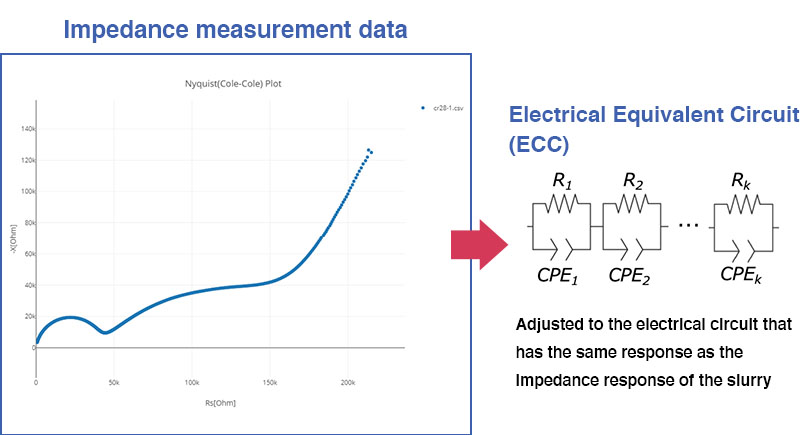# [LIB Electrode Slurry Analysis] How to Analyze Equivalent Circuit

## QHow are the parameters of the slurry’s electrical equivalent circuit calculated?

A

Since the impedance data for the slurry includes the characteristics of the electrical double-layer capacitance formed at the boundary of the electrode cell’s electrode pins and the slurry, the first step is to detect those characteristics and correct for them by including them in the slurry’s electrical equivalent circuit.
The circuit parameters can be calculated using the least squares method. The initial value is automatically input, and the parameters are optimized.
The parameters of the slurry’s electrical equivalent circuit are finalized when the coefficient of determination adjusted for degrees of freedom during parameter optimization is greater than or equal to a threshold value and the number of relaxation processes is minimized.
This threshold has been determined, through experience, based on impedance values for LIB electrode slurries as observed by Hioki. This approach makes it possible to seek agreement only, yielding an algorithm that limits the number of relaxation processes so as not to increase the number of non-physical parameters.

•Related Resources
- Principles of LIB Electrode Slurry Analysis: Slurry Analytical System